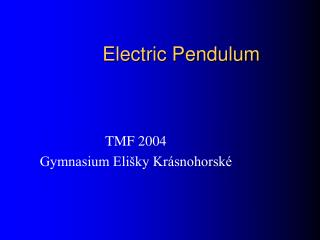DownloadDownload PresentationElectric Pendulum

# Electric Pendulum

Download Presentation## Electric Pendulum

- - - - - - - - - - - - - - - - - - - - - - - - - - - E N D - - - - - - - - - - - - - - - - - - - - - - - - - - -
##### Presentation Transcript

1. Electric Pendulum TMF 2004 Gymnasium Elišky Krásnohorské

2. Position of a ball Ball isn`t at beginning charged. The ball hangs at first: • vertically • slantingly (it doesn`t touch of capacitor) • slantingly (it touchs of capacitor)

3. The ball hangs vertically • No motion • Acting forces: • Gravity (Fg) • Reaction of a thread (Fr) • Resultant force is zero.

4. The ball hangs slantingly (it doesn`t touch of capacitor) • Matematic mechanic pendulum • Acting forces: • Gravity (Fg) • Reaction of a thread (Fr) • Friction • Resultant force isn`t zero (Fv). • Amplitude of oscillation will be decreased.

5. Dependences • Duration of an oscillation for small amplitude and small ball is: • For bigger ball we should include energy of rotation the ball. • For bigger amplitude we have to solve numerically. • l - lenght of pendulum • g - gravitational acceleration

6. The ball hangs slantingly (it touchs of capacitor) • Homogenous electric field. • Acting forces: • Gravity (Fg) • Reaction of a thread (Fr) • Electrostatic (Fe) • Friction • Resultant force isn`t zero (Fv). • Duration of an oscillation will be decreased – ball accelerated.

7. Electrostatic force • The size of electrostatic force is: • This force give energy to the ball and so the ball can accelerate. • r - radius of a ball •  - permitivity of enviroment • U - voltage on the capacitor • D - distance beetwen plates of capacitor

8. Special efects and measurement • Discharge of a ball by sparks and brush discharge. • The during of an oscillation was measured by an cassete recorder and sound balster on a computer.